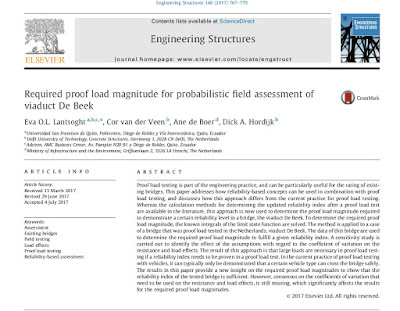## Tuesday, September 12, 2017

### Required proof load magnitude for probabilistic field assessment of viaduct De BeekWe recently published a paper in Engineering Structures, titled "Required proof load magnitude for probabilistic field assessment of viaduct De Beek". You can download the article for free through this link until October 7th 2017.

The abstract is as follows:

Proof load testing is part of the engineering practice, and can be particularly useful for the rating of existing bridges. This paper addresses how reliability-based concepts can be used in combination with proof load testing, and discusses how this approach differs from the current practice for proof load testing. Whereas the calculation methods for determining the updated reliability index after a proof load test are available in the literature, this approach is now used to determine the proof load magnitude required to demonstrate a certain reliability level in a bridge, the viaduct De Beek. To determine the required proof load magnitude, the known integrals of the limit state function are solved. The method is applied to a case of a bridge that was proof load tested in the Netherlands, viaduct De Beek. The data of this bridge are used to determine the required proof load magnitude to fulfill a given reliability index. A sensitivity study is carried out to identify the effect of the assumptions with regard to the coefficient of variation on the resistance and load effects. The result of this approach is that large loads are necessary in proof load testing if a reliability index needs to be proven in a proof load test. In the current practice of proof load testing with vehicles, it can typically only be demonstrated that a certain vehicle type can cross the bridge safely. The results in this paper provide a new insight on the required proof load magnitudes to show that the reliability index of the tested bridge is sufficient. However, consensus on the coefficients of variation that need to be used on the resistance and load effects, is still missing, which significantly affects the results for the required proof load magnitudes.

#### Post a Comment

Note: Only a member of this blog may post a comment.

UA-49678081-1